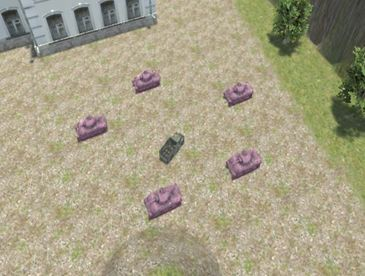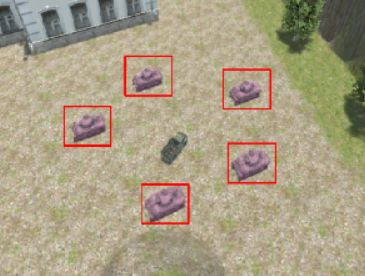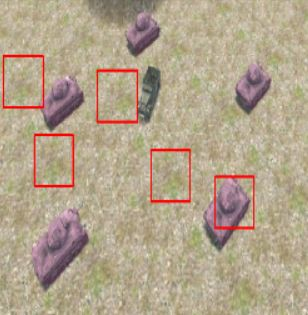# 機器學習實戰 | 簡單標的識別與意圖分析之模板匹配

（點選上方快速關註並設定為星標，一起學Python）

#### 初步標的識別``````tpl =cv.imread(r"D://PythonPicTemplate/pythinPIC/tankTemplate2.jpg")
cv.namedWindow('template image', cv.WINDOW_NORMAL)
cv.imshow("template image", tpl)
cv.namedWindow('target image', cv.WINDOW_NORMAL)
cv.imshow("target image", target)
#methods = [cv.TM_CCOEFF, cv.TM_CCOEFF_NORMED, cv.TM_CCORR,cv.TM_CCORR_NORMED, cv.TM_SQDIFF, cv.TM_SQDIFF_NORMED]
methods =[cv.TM_CCORR_NORMED ,cv.TM_CCORR_NORMED,cv.TM_CCORR_NORMED,cv.TM_CCORR_NORMED, cv.TM_CCORR_NORMED]
'''''''

'''``````

``````th, tw = tpl.shape[:2]
i =0
tl = []
br = []
for md in methods:
#print(md)
result = cv.matchTemplate(target, tpl, md)
min_val, max_val, min_loc, max_loc = cv.minMaxLoc(result)
if md == cv.TM_SQDIFF_NORMED:
tl.append(min_loc)
else:
tl.append(max_loc)
br.append((tl[i]+tw, tl[i]+th))
cv.rectangle(target, tl[i], br[i], (0, 0, 255), 2)
i += 1
cv.namedWindow("match-" + np.str(md), cv.WINDOW_NORMAL)
cv.imshow("match-" + np.str(md), target)``````#### 模板匹配的大致原理

1.模板的大小會影響匹配的精度；

2.幹擾現象嚴重，坦克藏在樹林裡很影響識別效果；

3.在實際運用中，模板的方向都必須要固定才能匹配成功；4.以上都還好說，最重要的是我都知道模板了，那我還寫程式碼在圖片裡識別個毛啊，對於這個課題直接圈出來不就行了。

GitHub：https://github.com/wangwei39120157028/Machine_Learning_research_on_simple_target_recognition_and_intention_analysis